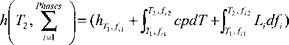## Heat Transfer Theory

For the moment we will assume that the velocity of the material points is zero. However, the arc is allowed to move with some velocity. Also an observer is allowed to move with some velocity. We will state the mathematics more precisely later but here we simply want to present an intuitive view of terms or mechanisms in the heat equation or conservation of energy. Within this fundamental principle there is a concept of a flux of energy with units of (W/m2). The flux q is proportional to thermal conductivity к ; a material

property, times the temperature gradient VT, i. e., q = - kVT. For k>0, this enforces the 2nd law of thermodynamics and the Clausien - Duhem inequality. There is the fundamental notion of energy per unit volume called specific enthalpy h (sometimes it is more convenient to use specific enthalpy per unit mass.). If there are no phase changes, then for a small change of temperature, the increment in specific enthalpy dh at constant pressure can often be expressed as dh = cp dT where с is a material property and dT is an increment in temperature.

More generally, the specific enthalpy at a given temperature and a set of і phases, with volume fraction f for phase і is given by the integral:However, in words, for a given temperature and a set of phase fractions the increment in specific enthalpy is the sum of increments due to temperature changes and increments due to latent heat generated by phase changes. The enthalpy is measured with respect to some chosen reference state. There can also be heat generated per unit volume Q with units of (W/m3). Most of the work of plastic deformation is converted to such heat source Q.

The heat equation expressed as a partial differential equation holds in the neighborhood of every point in a region of Euclidean space that mathematicians call a domain. On the boundaries of the domain, one must specify the interaction of the domain with the universe external to the domain. This is done by specifying boundary conditions. Basically, there are two choices for boundary conditions. One choice is to specify the flux on the boundary. This is the natural choice to describe the action of a burner or arc on a plate. Also if a section of the boundary is insulated so that the flux is zero, then specifying zero flux is natural. The other choice is to specify the temperature on the boundary. This is a natural choice for a plate partially immersed in an 'ice bath' with constant temperature. At each point on the boundary one must choose to specify either the flux or the temperature. For transient problems in which the temperature and phase fractions are functions of both space (x, y,z) and time t, one must state the conditions at time zero, t0 for every point in the domain. These are called initial conditions.

Now we can state the heat equation for a material point in the domain:

Dh

^ + V-(-xVr)+g = 0 (3-2)

The first term is the rate of change of specific enthalpy. The second term is the flow of heat out of or into the neighborhood of this point due to the flux. Mathematically it is the divergence of the flux V • q = V • (- kVT) (W/m2). The last term is Q the source of heat generation term with units of (W/m3).

One sees that there are only these three terms in the heat equation (with zero velocity) that can be 'manipulated' in addition to the associated boundary conditions of either prescribed flux or prescribed temperature type. Therefore any model of a weld heat source based on the heat equation must specify something about one or more terms in this heat equation or its BCs.

Suppose that a heat source is moving at a constant speed v in the positive x-direction. We can define an Eulerian (moving) frame with

origin at the center of the source, and coordinates (£ ,y, z) .The

transformation from (x, y, z) to (£ ,y, z) is given by: x - v t, where

t is time. Noting that the material time derivative T in equation (3-2) becomes:

r = 7:+|lT(=T,-vTt (3-3)

We obtain the equation of conservation of energy in the Eulerian frame:

T& + Туу +T~+T = + ^ (3'4)

Here, Tt represents the time derivative of temperature at a point

fixed with respect to the heat source. In a steady state, this derivative is zero, and so equation (3-4) becomes:

r«+r»+r*+f = - да7і (3-5>

When a and v are constant, equation (3-5) can be solved more easily by applying the transformation:

T = T0+e~^)l2<p^,y, z) (3-6)

Substituting this into equation (3-5), we have:

e(m)l2Q {ocv)2

<P% + + <Pa +--------- = <P (3-7)

к 4

rj2 (^^0*" (on■)/'’ Q

V q>-~——(p--eK ) —-Q 4 к

Equation (3-7) is symmetric positive definite. After properly transforming the boundary conditions from the T(0;,y, z) function to the (p(Z, y,z) function, we can easily solve this equation with a standard Lagrangian FEM code that contains a solver for positive definite symmetric matrix. Equation (3-7) applies only to linear systems (i. e. a is independent of J) because of equation (3-6).

Комментарии закрыты.